# Two implementations of heterodyne detection: direct heterodyne and as two homodyne measurements¶

Copyright (C) 2011 and later, Paul D. Nation & Robert J. Johansson

In :
%matplotlib inline

In :
import numpy as np
import scipy as sp

In :
import matplotlib.pyplot as plt

In :
from qutip import *


## Introduction¶

Homodyne and hetrodyne detection are techniques for measuring the quadratures of a field using photocounters. Homodyne detection (on-resonant) measures one quadrature and with heterodyne detection (off-resonant) both quadratures can be detected simulateously.

The evolution of a quantum system that is coupled to a field that is monitored with homodyne and heterodyne detector can be described with stochastic master equations. This notebook compares two different ways to implement the heterodyne detection stochastic master equation in QuTiP.

## Deterministic reference¶

In :
N = 15
w0 = 1.0 * 2 * np.pi
A = 0.1 * 2 * np.pi
times = np.linspace(0, 15, 301)
gamma = 0.25

ntraj = 150
nsubsteps = 50

a = destroy(N)
x = a + a.dag()
y = -1.0j*(a - a.dag())

H = w0 * a.dag() * a + A * (a + a.dag())

rho0 = coherent(N, np.sqrt(5.0), method='analytic')
c_ops = [np.sqrt(gamma) * a]
e_ops = [a.dag() * a, x, y]

In :
result_ref = mesolve(H, rho0, times, c_ops, e_ops)

In :
plot_expectation_values(result_ref);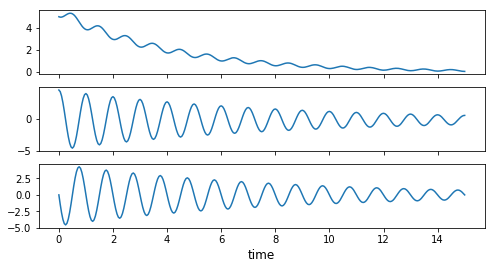## Heterodyne implementation #1¶

Stochastic master equation for heterodyne in Milburn's formulation

$\displaystyle d\rho(t) = -i[H, \rho(t)]dt + \gamma\mathcal{D}[a]\rho(t) dt + \frac{1}{\sqrt{2}} dW_1(t) \sqrt{\gamma} \mathcal{H}[a] \rho(t) + \frac{1}{\sqrt{2}} dW_2(t) \sqrt{\gamma} \mathcal{H}[-ia] \rho(t)$

where $\mathcal{D}$ is the standard Lindblad dissipator superoperator, and $\mathcal{H}$ is defined as above, and $dW_i(t)$ is a normal distributed increment with $E[dW_i(t)] = \sqrt{dt}$.

In QuTiP format we have:

$\displaystyle d\rho(t) = -i[H, \rho(t)]dt + D_{1}[A]\rho(t) dt + D_{2}^{(1)}[A]\rho(t) dW_1 + D_{2}^{(2)}[A]\rho(t) dW_2$

where $A = \sqrt{\gamma} a$, so we can identify

$\displaystyle D_{1}[A]\rho = \gamma \mathcal{D}[a]\rho = \mathcal{D}[A]\rho$

In :
from qutip.expect import expect_rho_vec

In :
L = liouvillian(H)
d1_operator = L + D
def d1_rho_func(t, rho_vec):
return d1_operator * rho_vec


$D_{2}^{(1)}[A]\rho = \frac{1}{\sqrt{2}} \sqrt{\gamma} \mathcal{H}[a] \rho = \frac{1}{\sqrt{2}} \mathcal{H}[A] \rho = \frac{1}{\sqrt{2}}(A\rho + \rho A^\dagger - \mathrm{Tr}[A\rho + \rho A^\dagger] \rho) \rightarrow \frac{1}{\sqrt{2}} \left\{(A_L + A_R^\dagger)\rho_v - \mathrm{Tr}[(A_L + A_R^\dagger)\rho_v] \rho_v\right\}$

$D_{2}^{(2)}[A]\rho = \frac{1}{\sqrt{2}} \sqrt{\gamma} \mathcal{H}[-ia] \rho = \frac{1}{\sqrt{2}} \mathcal{H}[-iA] \rho = \frac{-i}{\sqrt{2}}(A\rho - \rho A^\dagger - \mathrm{Tr}[A\rho - \rho A^\dagger] \rho) \rightarrow \frac{-i}{\sqrt{2}} \left\{(A_L - A_R^\dagger)\rho_v - \mathrm{Tr}[(A_L - A_R^\dagger)\rho_v] \rho_v\right\}$

In :
B1 = spre(c_ops) + spost(c_ops.dag())
B2 = spre(c_ops) + spost(c_ops.dag())
def d2_rho_func(t, rho_vec):
e1 = expect_rho_vec(B1.data, rho_vec, False)
drho1 = B1 * rho_vec - e1 * rho_vec

e1 = expect_rho_vec(B2.data, rho_vec, False)
drho2 = B2 * rho_vec - e1 * rho_vec

return np.vstack([1.0/np.sqrt(2) * drho1, -1.0j/np.sqrt(2) * drho2])


The heterodyne currents for the $x$ and $y$ quadratures are

$J_x(t) = \sqrt{\gamma}\left<x\right> + \sqrt{2} \xi(t)$

$J_y(t) = \sqrt{\gamma}\left<y\right> + \sqrt{2} \xi(t)$

where $\xi(t) = \frac{dW}{dt}$.

In qutip we define these measurement operators using the m_ops = [[x, y]] and the coefficients to the noise terms dW_factor = [sqrt(2/gamma), sqrt(2/gamma)].

In :
result = general_stochastic(ket2dm(rho0), times, d1_rho_func, d2_rho_func,
e_ops=[spre(op) for op in e_ops],
len_d2=2, ntraj=ntraj, nsubsteps=nsubsteps*2, solver="platen",
dW_factors=[np.sqrt(2/gamma), np.sqrt(2/gamma)],
m_ops=[spre(x), spre(y)],
store_measurement=True, map_func=parallel_map)

10.0%. Run time: 726.94s. Est. time left: 00:01:49:02
20.0%. Run time: 1177.90s. Est. time left: 00:01:18:31
30.0%. Run time: 1448.19s. Est. time left: 00:00:56:19
40.0%. Run time: 1744.10s. Est. time left: 00:00:43:36
50.0%. Run time: 2050.39s. Est. time left: 00:00:34:10
60.0%. Run time: 2446.47s. Est. time left: 00:00:27:10
70.0%. Run time: 3425.91s. Est. time left: 00:00:24:28
80.0%. Run time: 4123.43s. Est. time left: 00:00:17:10
90.0%. Run time: 4739.05s. Est. time left: 00:00:08:46
100.0%. Run time: 5331.43s. Est. time left: 00:00:00:00
Total run time: 5331.51s

In :
plot_expectation_values([result, result_ref]);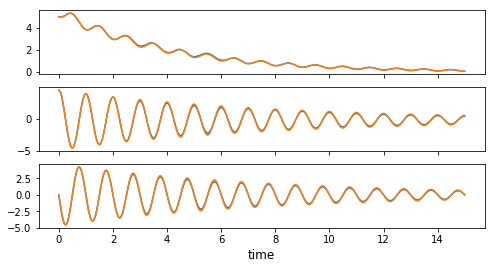In :
fig, ax = plt.subplots(figsize=(8,4))

for m in result.measurement:
ax.plot(times, m[:, 0].real, 'b', alpha=0.05)
ax.plot(times, m[:, 1].real, 'r', alpha=0.05)

ax.plot(times, result_ref.expect, 'b', lw=2);
ax.plot(times, result_ref.expect, 'r', lw=2);

ax.set_ylim(-10, 10)
ax.set_xlim(0, times.max())
ax.set_xlabel('time', fontsize=12)
ax.plot(times, np.array(result.measurement).mean(axis=0)[:,0].real, 'k', lw=2);
ax.plot(times, np.array(result.measurement).mean(axis=0)[:,1].real, 'k', lw=2);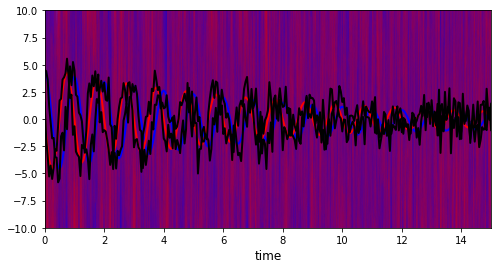## Heterodyne implementation #2: using two homodyne measurements¶

We can also write the heterodyne equation as

$\displaystyle d\rho(t) = -i[H, \rho(t)]dt + \frac{1}{2}\gamma\mathcal{D}[a]\rho(t) dt + \frac{1}{\sqrt{2}} dW_1(t) \sqrt{\gamma} \mathcal{H}[a] \rho(t) + \frac{1}{2}\gamma\mathcal{D}[a]\rho(t) dt + \frac{1}{\sqrt{2}} dW_2(t) \sqrt{\gamma} \mathcal{H}[-ia] \rho(t)$

And using the QuTiP format for two stochastic collapse operators, we have:

$\displaystyle d\rho(t) = -i[H, \rho(t)]dt + D_{1}[A_1]\rho(t) dt + D_{2}[A_1]\rho(t) dW_1 + D_{1}[A_2]\rho(t) dt + D_{2}[A_2]\rho(t) dW_2$

so we can also identify

$\displaystyle D_{1}[A_1]\rho = \frac{1}{2}\gamma \mathcal{D}[a]\rho = \mathcal{D}[\sqrt{\gamma}a/\sqrt{2}]\rho = \mathcal{D}[A_1]\rho$

$\displaystyle D_{1}[A_2]\rho = \frac{1}{2}\gamma \mathcal{D}[a]\rho = \mathcal{D}[-i\sqrt{\gamma}a/\sqrt{2}]\rho = \mathcal{D}[A_2]\rho$

$D_{2}[A_1]\rho = \frac{1}{\sqrt{2}} \sqrt{\gamma} \mathcal{H}[a] \rho = \mathcal{H}[A_1] \rho$

$D_{2}[A_2]\rho = \frac{1}{\sqrt{2}} \sqrt{\gamma} \mathcal{H}[-ia] \rho = \mathcal{H}[A_2] \rho$

where $A_1 = \sqrt{\gamma} a / \sqrt{2}$ and $A_2 = -i \sqrt{\gamma} a / \sqrt{2}$.

In summary we have

$\displaystyle d\rho(t) = -i[H, \rho(t)]dt + \sum_i\left\{\mathcal{D}[A_i]\rho(t) dt + \mathcal{H}[A_i]\rho(t) dW_i\right\}$

which is a simultaneous homodyne detection with $A_1 = \sqrt{\gamma}a/\sqrt{2}$ and $A_2 = -i\sqrt{\gamma}a/\sqrt{2}$

Here the two heterodyne currents for the $x$ and $y$ quadratures are

$J_x(t) = \sqrt{\gamma/2}\left<x\right> + \xi(t)$

$J_y(t) = \sqrt{\gamma/2}\left<y\right> + \xi(t)$

where $\xi(t) = \frac{dW}{dt}$.

In qutip we can use the predefined homodyne solver for solving this problem.

In :
opt = Options()
opt.store_states = True
result = smesolve(H, rho0, times, [], [np.sqrt(gamma/2) * a, -1.0j * np.sqrt(gamma/2) * a],
e_ops, ntraj=100, nsubsteps=nsubsteps, solver="taylor15",
m_ops=[x, y], dW_factors=[np.sqrt(2/gamma), np.sqrt(2/gamma)],
method='homodyne', store_measurement=True,
map_func=parallel_map)

10.0%. Run time:  66.22s. Est. time left: 00:00:09:55
20.0%. Run time: 131.95s. Est. time left: 00:00:08:47
30.0%. Run time: 195.57s. Est. time left: 00:00:07:36
40.0%. Run time: 257.92s. Est. time left: 00:00:06:26
50.0%. Run time: 324.28s. Est. time left: 00:00:05:24
60.0%. Run time: 393.51s. Est. time left: 00:00:04:22
70.0%. Run time: 465.37s. Est. time left: 00:00:03:19
80.0%. Run time: 533.48s. Est. time left: 00:00:02:13
90.0%. Run time: 597.27s. Est. time left: 00:00:01:06
100.0%. Run time: 672.84s. Est. time left: 00:00:00:00
Total run time: 672.92s

In :
plot_expectation_values([result, result_ref])

Out:
(<Figure size 576x288 with 3 Axes>,
array([[<matplotlib.axes._subplots.AxesSubplot object at 0x7fdeb0d370f0>],
[<matplotlib.axes._subplots.AxesSubplot object at 0x7fde9ee7b240>],
[<matplotlib.axes._subplots.AxesSubplot object at 0x7fdeb0d66940>]],
dtype=object))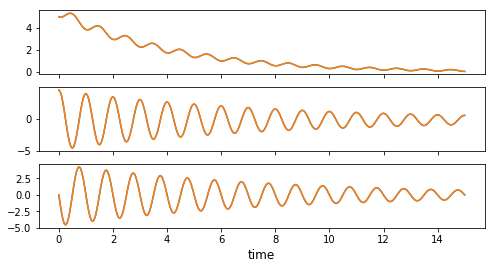In :
fig, ax = plt.subplots(figsize=(8,4))

for m in result.measurement:
ax.plot(times, m[:, 0].real, 'b', alpha=0.05)
ax.plot(times, m[:, 1].real, 'r', alpha=0.05)

ax.plot(times, result_ref.expect, 'b', lw=2);
ax.plot(times, result_ref.expect, 'r', lw=2);

ax.set_xlim(0, times.max())
ax.set_ylim(-25, 25)
ax.set_xlabel('time', fontsize=12)
ax.plot(times, np.array(result.measurement).mean(axis=0)[:,0].real, 'k', lw=2);
ax.plot(times, np.array(result.measurement).mean(axis=0)[:,1].real, 'k', lw=2);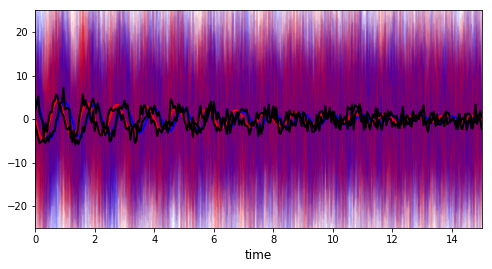## Implementation #3: builtin function for heterodyne¶

In :
result = smesolve(H, rho0, times, [], [np.sqrt(gamma) * a],
e_ops, ntraj=ntraj, nsubsteps=nsubsteps, solver="taylor15",
method='heterodyne', store_measurement=True,
map_func=parallel_map)

10.0%. Run time: 102.92s. Est. time left: 00:00:15:26
20.0%. Run time: 192.86s. Est. time left: 00:00:12:51
30.0%. Run time: 294.94s. Est. time left: 00:00:11:28
40.0%. Run time: 394.82s. Est. time left: 00:00:09:52
50.0%. Run time: 496.31s. Est. time left: 00:00:08:16
60.0%. Run time: 600.57s. Est. time left: 00:00:06:40
70.0%. Run time: 696.35s. Est. time left: 00:00:04:58
80.0%. Run time: 790.93s. Est. time left: 00:00:03:17
90.0%. Run time: 889.34s. Est. time left: 00:00:01:38
100.0%. Run time: 977.49s. Est. time left: 00:00:00:00
Total run time: 977.51s

In :
plot_expectation_values([result, result_ref]);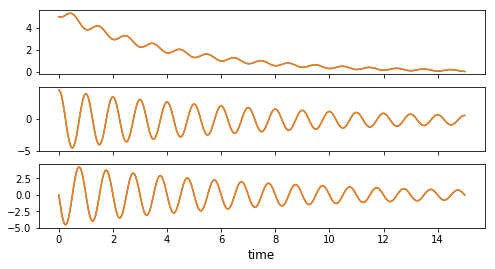In :
fig, ax = plt.subplots(figsize=(8,4))

for m in result.measurement:
ax.plot(times, m[:, 0, 0].real / np.sqrt(gamma), 'b', alpha=0.05)
ax.plot(times, m[:, 0, 1].real / np.sqrt(gamma), 'r', alpha=0.05)

ax.plot(times, result_ref.expect, 'b', lw=2);
ax.plot(times, result_ref.expect, 'r', lw=2);

ax.set_xlim(0, times.max())
ax.set_ylim(-15, 15)
ax.set_xlabel('time', fontsize=12)
ax.plot(times, np.array(result.measurement).mean(axis=0)[:, 0, 0].real / np.sqrt(gamma), 'k', lw=2);
ax.plot(times, np.array(result.measurement).mean(axis=0)[:, 0, 1].real / np.sqrt(gamma), 'k', lw=2);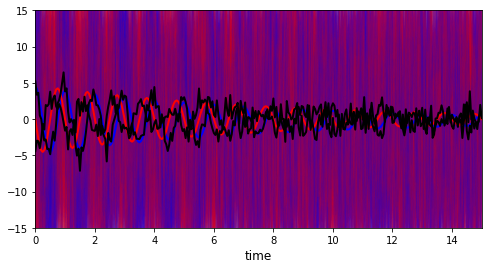## Common problem¶

For some systems, the resulting density matrix can become unphysical due to the accumulation of computation error.

In :
N = 5
w0 = 1.0 * 2 * np.pi
A = 0.1 * 2 * np.pi
times = np.linspace(0, 15, 301)
gamma = 0.25

ntraj = 150
nsubsteps = 50

a = destroy(N)
x = a + a.dag()
y = -1.0j*(a - a.dag())

H = w0 * a.dag() * a + A * (a + a.dag())

rho0 = coherent(N, np.sqrt(5.0), method='analytic')
c_ops = [np.sqrt(gamma) * a]
e_ops = [a.dag() * a, x, y]

opt = Options()
opt.store_states = True
result = smesolve(H, rho0, times, [], [np.sqrt(gamma) * a],
e_ops, ntraj=1, nsubsteps=5, solver="euler",
method='heterodyne', store_measurement=True,
map_func=parallel_map, options=opt, normalize=False)

Total run time:   0.22s

In :
result.states

Out:
Quantum object: dims = [, ], shape = (5, 5), type = oper, isherm = True\begin{equation*}\left(\begin{array}{*{11}c}2.207 & (5.321+4.567j) & (-11.562+21.772j) & (-44.949-22.307j) & (26.207-43.859j)\\(5.321-4.567j) & -1.071 & (1.224-2.188j) & (4.446+2.296j) & (-2.682+4.330j)\\(-11.562-21.772j) & (1.224+2.188j) & -0.172 & (-0.306-0.158j) & (0.194-0.303j)\\(-44.949+22.307j) & (4.446-2.296j) & (-0.306+0.158j) & 0.036 & (-0.012+0.018j)\\(26.207+43.859j) & (-2.682-4.330j) & (0.194+0.303j) & (-0.012-0.018j) & 0.001\\\end{array}\right)\end{equation*}
In :
sp.linalg.eigh(result.states.full())

Out:
(array([-3.14720041e-02, -6.10858380e-05,  1.14307166e-03,  1.18849700e-02,
3.23425775e-01]),
array([[-0.39567109-0.j        ,  0.63456229+0.j        ,
-0.62393366-0.j        , -0.18622189-0.j        ,
0.12962751-0.j        ],
[ 0.55963914-0.01726392j,  0.62575152-0.1820371j ,
0.13269909-0.21765968j,  0.3300729 +0.16800435j,
-0.24210188+0.03212229j],
[-0.37291891-0.05788453j,  0.02981755-0.32786487j,
0.36972653-0.45755152j, -0.26768387+0.56384386j,
0.11079367+0.03599329j],
[ 0.23987807-0.06051551j, -0.2060353 -0.09136364j,
-0.33745337-0.15310173j, -0.50878937+0.1069029j ,
-0.61438491-0.32081137j],
[ 0.23004721-0.52288232j, -0.04433998+0.10714422j,
-0.12459429+0.2164076j ,  0.07332225+0.40361154j,
0.42487235-0.49907466j]]))

Using smaller integration steps by increasing the nsubstep will lower the numerical errors.
The solver algorithm used affect the convergence and numerical error. Notable solvers are:

• euler: order 0.5 fastest, but lowest order. Only solver that accept non-commuting sc_ops
• rouchon: order 1.0?, build to keep the density matrix physical
• taylor1.5: order 1.5, default solver, reasonably fast for good convergence.
• taylor2.0: order 2.0, even better convergence but can only take 1 homodyne sc_ops.

To list list all available solver, use help(stochastic_solvers)

In :
help(stochastic_solvers)

Help on function stochastic_solvers in module qutip.stochastic:

stochastic_solvers()
Available solvers for ssesolve and smesolve
euler-maruyama:
A simple generalization of the Euler method for ordinary
differential equations to stochastic differential equations.
Only solver which could take non-commuting sc_ops. *not tested*
-Order 0.5
-Code: 'euler-maruyama', 'euler', 0.5

milstein, Order 1.0 strong Taylor scheme:
Better approximate numerical solution to stochastic
differential equations.
-Order strong 1.0
-Code: 'milstein', 1.0
Numerical Solution of Stochastic Differential Equations
Chapter 10.3 Eq. (3.1), By Peter E. Kloeden, Eckhard Platen

milstein-imp, Order 1.0 implicit strong Taylor scheme:
Implicit milstein scheme for the numerical simulation of stiff
stochastic differential equations.
-Order strong 1.0
-Code: 'milstein-imp'
Numerical Solution of Stochastic Differential Equations
Chapter 12.2 Eq. (2.9), By Peter E. Kloeden, Eckhard Platen

predictor-corrector:
Generalization of the trapezoidal method to stochastic
differential equations. More stable than explicit methods.
-Order strong 0.5, weak 1.0
Only the stochastic part is corrected.
(alpha = 0, eta = 1/2)
-Code: 'pred-corr', 'predictor-corrector', 'pc-euler'
Both the deterministic and stochastic part corrected.
(alpha = 1/2, eta = 1/2)
-Code: 'pc-euler-imp', 'pc-euler-2', 'pred-corr-2'
Numerical Solution of Stochastic Differential Equations
Chapter 15.5 Eq. (5.4), By Peter E. Kloeden, Eckhard Platen

platen:
Explicit scheme, create the milstein using finite difference instead of
derivatives. Also contain some higher order terms, thus converge better
than milstein while staying strong order 1.0.
Do not require derivatives, therefore usable for
:func:qutip.stochastic.general_stochastic
-Order strong 1.0, weak 2.0
-Code: 'platen', 'platen1', 'explicit1'
The Theory of Open Quantum Systems
Chapter 7 Eq. (7.47), H.-P Breuer, F. Petruccione

rouchon:
Scheme keeping the positivity of the density matrix. (smesolve only)
-Order strong 1.0?
-Code: 'rouchon', 'Rouchon'
Efficient Quantum Filtering for Quantum Feedback Control
Pierre Rouchon, Jason F. Ralph
arXiv:1410.5345 [quant-ph]

taylor1.5, Order 1.5 strong Taylor scheme:
Solver with more terms of the Ito-Taylor expansion.
Default solver for smesolve and ssesolve.
-Order strong 1.5
-Code: 'taylor1.5', 'taylor15', 1.5, None
Numerical Solution of Stochastic Differential Equations
Chapter 10.4 Eq. (4.6), By Peter E. Kloeden, Eckhard Platen

taylor1.5-imp, Order 1.5 implicit strong Taylor scheme:
implicit Taylor 1.5 (alpha = 1/2, beta = doesn't matter)
-Order strong 1.5
-Code: 'taylor1.5-imp', 'taylor15-imp'
Numerical Solution of Stochastic Differential Equations
Chapter 12.2 Eq. (2.18), By Peter E. Kloeden, Eckhard Platen

explicit1.5, Explicit Order 1.5 Strong Schemes:
Reproduce the order 1.5 strong Taylor scheme using finite difference
instead of derivatives. Slower than taylor15 but usable by
:func:qutip.stochastic.general_stochastic
-Order strong 1.5
-Code: 'explicit1.5', 'explicit15', 'platen15'
Numerical Solution of Stochastic Differential Equations
Chapter 11.2 Eq. (2.13), By Peter E. Kloeden, Eckhard Platen

taylor2.0, Order 2 strong Taylor scheme:
Solver with more terms of the Stratonovich expansion.
-Order strong 2.0
-Code: 'taylor2.0', 'taylor20', 2.0
Numerical Solution of Stochastic Differential Equations
Chapter 10.5 Eq. (5.2), By Peter E. Kloeden, Eckhard Platen

---All solvers, except taylor2.0, are usable in both smesolve and ssesolve
and for both heterodyne and homodyne. taylor2.0 only work for 1 stochastic
operator not dependent of time with the homodyne method.
The :func:qutip.stochastic.general_stochastic only accept derivatives
free solvers: ['euler', 'platen', 'explicit1.5'].

Available solver for photocurrent_sesolve and photocurrent_mesolve:
Photocurrent use ordinary differential equations between
stochastic "jump/collapse".
euler:
Euler method for ordinary differential equations.
Default solver
-Order 1.0
-Code: 'euler'

predictor–corrector:
predictor–corrector method (PECE) for ordinary differential equations.
-Order 2.0
-Code: 'pred-corr'



## Versions¶

In :
from qutip.ipynbtools import version_table

version_table()

Out:
SoftwareVersion
QuTiP4.4.0.dev0+ea5344d9
Numpy1.16.0
SciPy1.2.0
matplotlib3.0.2
Cython0.29.3
Number of CPUs1
BLAS InfoOPENBLAS
IPython7.2.0
Python3.6.7 (default, Oct 22 2018, 11:32:17) [GCC 8.2.0]
OSposix [linux]
Wed Jan 30 13:43:39 2019 JST